# Linear Modeling - A Maximum Likelihood Approach

## ERRORS AS NOISE

Explicitly model the noise (the errors between the model and the observations) in the data by incorporating a random variable.

### Advantages of incorporating a noise term into our model:

• Allows us to express the level of uncertainty in our estimate of the model parameters, $w$
• Provides several benefits over loss minimisation.
• Able to quantify the uncertainty in our parameters and the ability to express uncertainties in our predictions(just use the available).

### How to present linear model that fit our data

For linear model, we can add a variable to think in a generative way. $t _ { n } = \mathbf { w } ^ { T } \mathbf { x } _ { n } + \epsilon _ { n }$ $\epsilon _ { n }$ is continuous random variable

also, $\epsilon _ { n }$ should be independent, which means: $p \left( \epsilon _ { 1 } , \ldots , \epsilon _ { N } \right) = \prod _ { n = 1 } ^ { N } p \left( \epsilon _ { n } \right)$. Usually, we use gaussian distribution: $p ( \epsilon ) = \mathcal { N } \left( \mu , \sigma ^ { 2 } \right)$

mu, sigma = 0, 0.1 # mean and standard deviation
s = np.random.normal(mu, sigma, 1000)
print(abs(mu - np.mean(s)) < 0.01)
print(abs(sigma - np.std(s, ddof=1)) < 0.01)
import matplotlib.pyplot as plt
count, bins, ignored = plt.hist(s, 30, density=True)
plt.plot(bins, 1/(sigma * np.sqrt(2 * np.pi)) * np.exp( - (bins - mu)**2 / (2 * sigma**2) ),linewidth=2, color='r')
plt.show()
##out
#true
#true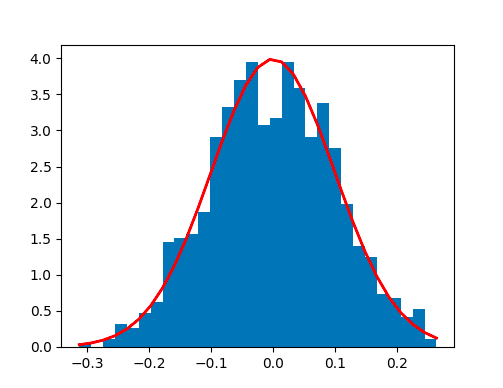Guassian Distribution

import numpy as np
mu, sigma = 0, 0.05 # mean and standard deviation
s = np.random.normal(mu, sigma, 10)
x = np.vstack(np.linspace(0,9,num=10))
w = np.array([-0.05,9.375])
xx = np.hstack((x,np.ones_like(x)))
y = xx @ w + s
plt.scatter(x.T,y)
plt.show()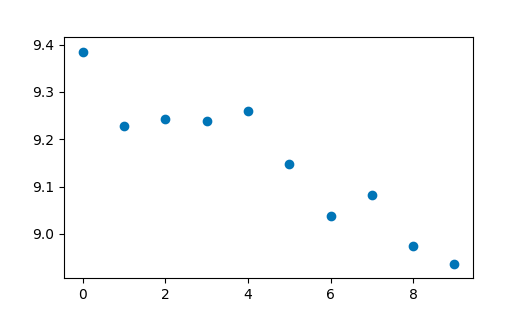Linear sample

### Dataset likelihood

Usually we are not interested in the likelihood of a single data point but that of all of the data, like:

$p \left( t _ { 1 } , \ldots , t _ { N } | \mathbf { x } _ { 1 } , \ldots , \mathbf { x } _ { N } , \mathbf { w } , \sigma ^ { 2 } \right)$ for short $p ( \mathbf { t } | \mathbf { X } , \mathbf { w } , \sigma ^ { 2 } )$

We assume $p$ is gaussian distribution, and noise at each point is completely independent, then
$L = p ( \mathbf { t } | \mathbf { X } , \mathbf { w } , \sigma ^ { 2 } ) = \prod _ { n = 1 } ^ { N } p \left( t _ { n } | \mathbf { x } _ { n } , \mathbf { w } , \sigma ^ { 2 } \right) = \prod _ { n = 1 } ^ { N } \mathcal { N } \left( \mathbf { w } ^ { \top } \mathbf { x } _ { n } , \sigma ^ { 2 } \right)$ (*)

$t _{n}$ are conditionally independent – given a value for $w$, the $t _{n}$ are independent Eg: To get $p \left( t _ { 1 } 960 | \mathbf { x } _ { 1960 } , \mathbf { X } , \mathbf { t } \right)$

we can have $p \left( t _ { 1960 } | \mathbf { x } _ { 1960 } , \mathbf { X } , \mathbf { t } \right) = \frac { p \left( t _ { 1960 } , \mathbf { t } | \mathbf { x } _ { 1960 } , \mathbf { X } \right) } { p ( \mathbf { t } | \mathbf { X } ) }$

then\ $p \left( t _ { 1960 } | \mathbf { x } _ { 1960 } , \mathbf { X } , \mathbf { t } \right) = \frac { p \left( t _ { 1960 } | \mathbf { x } _ { 1060 } \right) \prod _ { n } p \left( t _ { n } | \mathbf { x } _ { n } \right) } { \prod _ { n } p \left( t _ { n } | \mathbf { x } _ { n } \right) } = p \left( t _ { 1960 } | \mathbf { x } _ { 1960 } \right)$

that shows in our model, $t _{n}$ are conditionally independent – given a value for $w$, the $t _{n}$ are independent. This dependence is encapsulated in the parameter $w$. In a word, if we know $w$, all that remains is the errors between the observed data and $w ^{T}x_{n}$, errors are independent(noise–gaussian distribution),conditioned on w, the observations are independent. Without a model (and therefore a w), the observations are not independent.

### Maximum likelihood

#### Why we need to maximum the likelihood?

Because we have a fixed dataset, varying the model will result in different likelihood values, so a sensible choice of model would be that which maximised the likelihood ==> choose the $w$ and $\sigma ^{2}$ to maximum $L$.

\begin{aligned} \log L & = \sum _ { n = 1 } ^ { N } \log \left( \frac { 1 } { \sqrt { 2 \pi \sigma ^ { 2 } } } \exp \left\{ - \frac { 1 } { 2 \sigma ^ { 2 } } \left( t _ { n } - f \left( \mathbf { x } _ { n } ; \mathbf { w } \right) \right) ^ { 2 } \right\} \right) \\ & = \sum _ { n = 1 } ^ { N } \left( - \frac { 1 } { 2 } \log ( 2 \pi ) - \log \sigma - \frac { 1 } { 2 \sigma ^ { 2 } } \left( t _ { n } - f \left( \mathbf { x } _ { n } , \mathbf { w } \right) \right) ^ { 2 } \right) \\ & = - \frac { N } { 2 } \log 2 \pi - N \log \sigma - \frac { 1 } { 2 \sigma ^ { 2 } } \sum _ { n = 1 } ^ { N } \left( t _ { n } - f \left( \mathbf { x } _ { n } ; \mathbf { w } \right) \right) ^ { 2 } \end{aligned}

We know that $logL$ is a multivariable function that looks like:$f(w,\sigma)$, so we can deriviate $logL$ to find $w,\sigma$ corresponding to the maximum $logL$

\begin{aligned} \frac { \partial \log L } { \partial \mathbf { w } } & = \frac { 1 } { \sigma ^ { 2 } } \sum _ { n = 1 } ^ { N } \mathbf { x } _ { n } \left( t _ { n } - \mathbf { x } _ { n } ^ { \top } \mathbf { w } \right) \\ & = \frac { 1 } { \sigma ^ { 2 } } \sum _ { n = 1 } ^ { N } \mathbf { x } _ { n } t _ { n } - \mathbf { x } _ { n } \mathbf { x } _ { n } ^ { \top } \mathbf { w } = \mathbf { 0 } \end{aligned}

$\mathbf { X } = \left[ \begin{array} { c } { \mathbf { x } _ { 1 } ^ { \top } } \\ { \mathbf { x } _ { 2 } ^ { \top } } \\ { \vdots } \\ { \mathbf { x } _ { N } ^ { \top } } \end{array} \right] = \left[ \begin{array} { c c } { 1 } & { x _ { 1 } } \\ { 1 } & { x _ { 2 } } \\ { \vdots } & { \vdots } \\ { 1 } & { x _ { N } } \end{array} \right] , \quad \mathbf { t } = \left[ \begin{array} { c } { t _ { 1 } } \\ { t _ { 2 } } \\ { \vdots } \\ { t _ { N } } \end{array} \right]$

$\sum _ { n = 1 } ^ { N } \mathbf { x } _ { n } t _ { n }$ can be write as $X ^ {T}t$ and $\sum _ { n = 1 } ^ { N } \mathbf { x } _ { n } \mathbf { x } _ { n } ^ { \top } \mathbf { w }$ can be write as $X^{T}Xw$ then\

\begin{aligned}\frac { \partial \log L } { \partial \mathbf { w } } = \frac { 1 } { \sigma ^ { 2 } } \left( \mathbf { X } ^ { \top } \mathbf { t } - \mathbf { X } ^ { \top } \mathbf { X } \mathbf { w } \right) = \mathbf { 0 }\end{aligned} $\Rightarrow$ $\mathbf { w } = \left( \mathbf { X } ^ { \top } \mathbf { X } \right) ^ { - 1 } \mathbf { X } ^ { \top } \mathbf { t }$ the same as the result we got in the previous chapter.

Also for $\sigma$
$\frac { \partial \log L } { \partial \sigma } = - \frac { N } { \sigma } + \frac { 1 } { \sigma ^ { 3 } } \sum _ { n = 1 } ^ { N } \left( t _ { n } - \mathbf { x } ^ { \top } \widehat { \mathbf { w } } \right) ^ { 2 } = 0$
\begin{aligned} \sigma ^ { 2 } & = \frac { 1 } { N } ( \mathbf { t } - \mathbf { X } \widehat { \mathbf { w } } ) ^ { \top } ( \mathbf { t } - \mathbf { X } \widehat { \mathbf { w } } ) \\ & = \frac { 1 } { N } \left( \mathbf { t } ^ { \top } \mathbf { t } - 2 \mathbf { t } ^ { \top } \mathbf { X } \widehat { \mathbf { w } } + \widehat { \mathbf { w } } ^ { \top } \mathbf { X } ^ { \top } \mathbf { X } \widehat { \mathbf { w } } \right) \end{aligned}
\begin{aligned} \widehat { \sigma ^ { 2 } } & = \frac { 1 } { N } \left( \mathbf { t } ^ { \top } \mathbf { t } - 2 \mathbf { t } ^ { \top } \mathbf { X } \left( \mathbf { X } ^ { \top } \mathbf { X } \right) ^ { - 1 } \mathbf { X } ^ { \top } \mathbf { t } + \mathbf { t } ^ { \top } \mathbf { X } \left( \mathbf { X } ^ { \top } \mathbf { X } \right) ^ { - 1 } \mathbf { X } ^ { \top } \mathbf { X } \left( \mathbf { X } ^ { \top } \mathbf { X } \right) ^ { - 1 } \mathbf { X } ^ { \top } \mathbf { t } \right) \\ & = \frac { 1 } { N } \left( \mathbf { t } ^ { \top } \mathbf { t } - 2 \mathbf { t } ^ { \top } \mathbf { X } \left( \mathbf { X } ^ { \top } \mathbf { X } \right) ^ { - 1 } \mathbf { X } ^ { \top } \mathbf { t } + \mathbf { t } ^ { \top } \mathbf { X } \left( \mathbf { X } ^ { \top } \mathbf { X } \right) ^ { - 1 } \mathbf { X } ^ { \top } \mathbf { t } \right) \\ & = \frac { 1 } { N } \left( \mathbf { t } ^ { \top } \mathbf { t } - \mathbf { t } ^ { \top } \mathbf { X } \left( \mathbf { X } ^ { \top } \mathbf { X } \right) ^ { - 1 } \mathbf { X } ^ { \top } \mathbf { t } \right) \end{aligned}

import pylab as plt
import urllib.request
urllib.request.urlretrieve('https://raw.githubusercontent.com/sdrogers/fcmlcode/master/notebooks/data/olympic100m.txt', 'olympic100m.txt')
import numpy as np
import math
x = data[:,0][:,None]
t = data[:,1][:,None]
X = np.hstack((np.ones_like(x),x))  # we just add more to here to change all
X = np.asmatrix(X)
t = t # This is already a vector!
w = (X.T*X).I*X.T*t
delta = (1.0/(len(x)-1)) * (t.T @ t - t.T @ X @ w)  # unbaised
#output
w = [36.4, -1.33*e-2]
delta = 0.052


$\widehat { \sigma ^ { 2 } } = \frac { 1 } { N } \left( \mathbf { t } ^ { \top } \mathbf { t } - \mathbf { t } ^ { \top } \mathbf { X } \widehat { \mathbf { w } } \right)$

But!!! To ensure we have found the maximum, the hessian matrix should be negative definite $\mathbf { H } = \left[ \begin{array} { c c c c } { \frac { \partial ^ { 2 } f } { \partial w _ { 1 } ^ { 2 } } } & { \frac { \partial ^ { 2 } f } { \partial w _ { 1 } \partial w _ { 2 } } } & { \cdots } & { \frac { \partial ^ { 2 } f } { \partial w _ { 1 } \partial w _ { K } } } \\ { \frac { \partial ^ { 2 } f } { \partial w _ { 2 } \partial w _ { 1 } } } & { \frac { \partial ^ { 2 } f } { \partial w _ { 2 } ^ { 2 } } } & { \cdots } & { \frac { \partial ^ { 2 } f } { \partial w _ { 2 } \partial w _ { K } } } \\ { \vdots } & { \vdots } & { \ddots } & { \vdots } \\ { \frac { \partial ^ { 2 } f } { \partial w _ { K } \partial w _ { 1 } } } & { \frac { \partial ^ { 2 } f } { \partial w _ { K } \partial w _ { 2 } } } & { \cdots } & { \frac { \partial ^ { 2 } f } { \partial w _ { K } ^ { 2 } } } \end{array} \right]$

The Hessian matrix of second-order partial derivatives can be computed by differentiating $\frac { \partial \log L } { \partial w }$ with respect to $w^{T}$:

$\frac { \partial ^ { 2 } \log L } { \partial \mathbf { w } \partial \mathbf { w } ^ { \top } } = - \frac { 1 } { \sigma ^ { 2 } } \mathbf { X } ^ { \top } \mathbf { X }$

#calculate Hessian matrix
import pylab as plt
import urllib.request
urllib.request.urlretrieve('https://raw.githubusercontent.com/sdrogers/fcmlcode/master/notebooks/data/olympic100m.txt', 'olympic100m.txt')
import numpy as np
import math
x = data[:,0][:,None]
t = data[:,1][:,None]
hx = np.sin((x-2660)/4.3)

X = np.hstack((np.ones_like(x),x))  # we just add more to here to change all
X = np.asmatrix(X)
t = t # This is already a vector!

w = (X.T*X).I*X.T*t
delta = (1.0/(len(x)-1)) * (t.T @ t - t.T @ X @ w)

H = -1.0/(delta[0,0]**2) * X.T @ X


for any vector $z$, we can see that $\mathbf { z } ^ { \top } \mathbf { X } ^ { \top } \mathbf { X } \mathbf { z } > 0$. because $Xz$ is a vector

Also, for $\widehat { \sigma ^ { 2 } }$,

$\frac { \partial ^ { 2 } \log L } { \partial \sigma ^ { 2 } } = \frac { N } { \sigma ^ { 2 } } - \frac { 3 } { \sigma ^ { 4 } } ( \mathbf { t } - \mathbf { X } \widehat { \mathbf { w } } ) ^ { \top } ( \mathbf { t } - \mathbf { X } \widehat { \mathbf { w } } )$

\begin{aligned} \frac { \partial ^ { 2 } \log L } { \partial \sigma ^ { 2 } } & = \frac { N } { \widehat { \sigma } ^ { 2 } } - \frac { 3 } { \left( \widehat { \sigma ^ { 2 } } \right) ^ { 2 } } N \widehat { \sigma ^ { 2 } } \\ & = - \frac { 2 N } { \widehat { \sigma } ^ { 2 } } \end{aligned} < 0 $\Rightarrow$ $\widehat { \sigma ^ { 2 } }$ corresponds to a maximum.

### Maximum likelihood favours complex models

#### Why?

\begin{aligned} \log L & = - \frac { N } { 2 } \log 2 \pi - \frac { N } { 2 } \log \widehat { \sigma ^ { 2 } } - \frac { 1 } { 2 \widehat { \sigma ^ { 2 } } } N \widehat { \sigma ^ { 2 } } \\ & = - \frac { N } { 2 } ( 1 + \log 2 \pi ) - \frac { N } { 2 } \log \widehat { \sigma ^ { 2 } } \end{aligned} that means if we decrease $\widehat { \sigma ^ { 2 } }$, we can get a bigger $L$, which means we have a more complex model. The more complex the model is, the more likely it is, if we were to use log L to help choose which particular model to use, it would always point us to models of increasing complexity !!! But in real world, it’s obviously not this case. In this situation, we need to trade off beween generalisation and over-fitting(bias-variance trade-off).

#### How to solve this problem?

• regularisation
• use prior distribution on the parameter values
• $\overline { \mathcal { M } } = \mathcal { B } ^ { 2 } + \mathcal { V }$, bias $\mathcal {B}$, and variance $\mathcal {V}$
• a model that is too simple will have a high bias(underfitting)
• a model that is too complex will have a high variance

### Effect of noise on parameter estimates

#### Uncertainty is estimated

$\widehat {w }$ is strongly influenced by the particular noise in the data $\Rightarrow$ it’s useful to know how much uncertainty there was in $\widehat { w }$, in general, we can calculate the expectation($\mathbf { E } _ { p ( \mathbf { t } | \mathbf { X } , \mathbf { w } , \sigma ^ { 2 } ) } \{ \widehat { \mathbf { w } } \}$) to show it’s uncertainty–given the result distribution, how it’s likely that $\hat {w}$

We already know: $p ( \mathbf { t } | \mathbf { X } , \mathbf { w } , \sigma ^ { 2 } ) = \prod _ { n = 1 } ^ { N } p \left( t _ { n } | \mathbf { x } _ { n } \mathbf { w } , \sigma ^ { 2 } \right) = \prod _ { n = 1 } ^ { N } \mathcal { N } \left( \mathbf { w } ^ { \top } \mathbf { x } _ { n } , \sigma ^ { 2 } \right)$

then\ $p ( \mathbf { t } | \mathbf { X } , \mathbf { w } , \sigma ^ { 2 } ) = \mathcal { N } \left( \mathbf { X } \mathbf { w } , \sigma ^ { 2 } \mathbf { I } \right)$

$\Rightarrow$ $\mathbf { E } _ { p ( \mathbf { t } | \mathbf { X } , \mathbf { w } , \sigma ^ { 2 } ) } \{ \widehat { \mathbf { w } } \} = \int \widehat { \mathbf { w } } p ( \mathbf { t } | \mathbf { X } , \mathbf { w } , \sigma ^ { 2 } ) d \mathbf { t }$ and $\widehat { \mathbf { w } } = \left( \mathbf { X } ^ { \top } \mathbf { X } \right) ^ { - 1 } \mathbf { X } ^ { \top } \mathbf { t }$ $\Rightarrow$ $\mathbf { E } _ { p ( \mathbf { t } | \mathbf { X } , \mathbf { w } , \sigma ^ { 2 } ) } \{ \widehat { \mathbf { w } } \}$ \begin{aligned} & = \left( \mathbf { X } ^ { \top } \mathbf { X } \right) ^ { - 1 } \mathbf { X } ^ { \top } \int \mathbf { t } p ( \mathbf { t } | \mathbf { X } , \mathbf { w } , \sigma ^ { 2 } ) d t \\ & = \left( \mathbf { X } ^ { \top } \mathbf { X } \right) ^ { - 1 } \mathbf { X } ^ { \top } \mathbf { E } _ { p ( \mathbf { t } | \mathbf { X } , \mathbf { w } , \sigma ^ { 2 } ) } \{ \mathbf { t } \} \\ & = \left( \mathbf { X } ^ { \top } \mathbf { X } \right) ^ { - 1 } \mathbf { X } ^ { \top } \mathbf { X } \mathbf { w } \\ & = \mathbf { w } \end{aligned} That means our estimation to $\hat {w}$ is unbiased.

What covariance matrix can represent:

• diagonal elements: parameters variability
• how parameters co-vary Eg: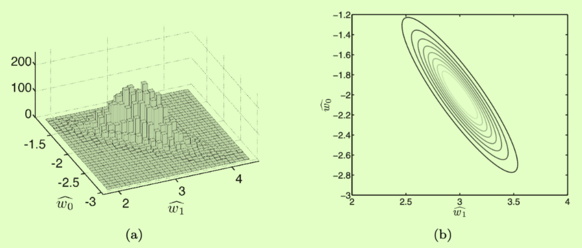Likelihood function In the picture above, increasing $\mathit{w1}$ causes a decrease in $w _{0}$ so we might expect the off-diagonal elements in the covariance matrix to be negative.

Cov $p ( \mathbf { t } | \mathbf { X } , \mathbf { w } , \sigma ^ { 2 } ) = \mathcal { N } \left( \mathbf { X } \mathbf { w } , \sigma ^ { 2 } \mathbf { I } \right)$ $\Rightarrow$ covariance of $t$ is $\sigma ^ { 2 } \mathbf { I }$ and its mean is $Xw$.

\begin{aligned} \operatorname { cov } \{ \widehat { \mathbf { w } } \} & = \mathbf { E } _ { p ( \mathbf { t } | \mathbf { X } , \mathbf { w } , \sigma ^ { 2 } ) } \left\{ \widehat { \mathbf { w } } \widehat { \mathbf { w } } ^ { \top } \right\} - \mathbf { E } _ { p ( \mathbf { t } | \mathbf { x } , \mathbf { w } , \sigma ^ { 2 } ) } \{ \widehat { \mathbf { w } } \} \mathbf { E } _ { p ( \mathbf { t } | \mathbf { X } , \mathbf { w } , \sigma ^ { 2 } ) } \{ \widehat { \mathbf { w } } \} ^ { \top } \\ & = \mathbf { E } _ { p ( \mathbf { t } | \mathbf { X } , \mathbf { w } , \sigma ^ { 2 } ) } \left\{ \widehat { \mathbf { w } } \widehat { \mathbf { w } } ^ { \top } \right\} - \mathbf { w } \mathbf { w } ^ { \top } \end{aligned}

$\mathbf { E } _ { p ( \mathbf { t } | \mathbf { X } , \mathbf { w } , \sigma ^ { 2 } ) } \left\{ \widehat { \mathbf { w } } \widehat { \mathbf { w } } ^ { \top } \right\} = \mathbf { E } _ { p ( \mathbf { t } | \mathbf { X } , \mathbf { w } , \sigma ^ { 2 } ) } \left \{ \left( \mathbf { X } ^ { \top } \mathbf { X } \right) ^ { - 1 } \mathbf { X } ^ { \top } \mathbf { t } \right) \left( \left( \mathbf { X } ^ { \top } \mathbf { X } \right) ^ { - 1 } \mathbf { X } ^ { \top } \mathbf { t } \right) ^ { \top } \}$ $= \left( \mathbf { X } ^ { \top } \mathbf { X } \right) ^ { - 1 } \mathbf { X } ^ { \top } \mathbf { E } _ { p ( \mathbf { t } | \mathbf { X } , \mathbf { w } , \sigma ^ { 2 } ) } \left\{ \mathbf { t } \mathbf { t } ^ { \top } \right\} \mathbf { X } \left( \mathbf { X } ^ { \top } \mathbf { X } \right) ^ { - 1 }$ $\operatorname { cov } \{ \mathbf { t } \} = \sigma ^ { 2 } \mathbf { I } = \mathbf { E } _ { p ( \mathbf { t } | \mathbf { X } , \mathbf { w } , \sigma ^ { 2 } ) } \left\{ \mathbf { t } \mathbf { t } ^ { \top } \right\} - \mathbf { E } _ { p ( \mathbf { t } | \mathbf { X } , \mathbf { w } , \sigma ^ { 2 } ) } \{ \mathbf { t } \} \mathbf { E } _ { p ( \mathbf { t } | \mathbf { X } , \mathbf { w } , \sigma ^ { 2 } ) } \{ \mathbf { t } \} ^ { \top }$

then:
\begin{aligned} \mathbf { E } _ { p ( \mathbf { t } | \mathbf { X } , \mathbf { w } , \sigma ^ { 2 } ) } \left\{ \widehat { \mathbf { w } } \widehat { \mathbf { w } } ^ { \top } \right\} = & \left( \mathbf { X } ^ { \top } \mathbf { X } \right) ^ { - 1 } \mathbf { X } ^ { \top } \mathbf { X } \mathbf { w } ^ { \top } \mathbf { X } ^ { \top } \mathbf { X } \left( \mathbf { X } ^ { \top } \mathbf { X } \right) ^ { - 1 } \\ & + \sigma ^ { 2 } \left( \mathbf { X } ^ { \top } \mathbf { X } \right) ^ { - 1 } \mathbf { X } ^ { \top } \mathbf { X } \left( \mathbf { X } ^ { \top } \mathbf { X } \right) ^ { - 1 } \end{aligned} $= \quad \mathbf { w } \mathbf{ w } ^ { T } + \sigma ^ { 2 } \left( \mathbf { X } ^ { \top } \mathbf { X } \right) ^ { - 1 }$ \begin{aligned} \operatorname { cov } \{ \widehat { \mathbf { w } } \} & = \mathbf { w } \mathbf { w } ^ { \top } + \sigma ^ { 2 } \left( \mathbf { X } ^ { \top } \mathbf { X } \right) ^ { - 1 } - \mathbf { w } \mathbf { w } ^ { \top } \\ & = \sigma ^ { 2 } \left( \mathbf { X } ^ { \top } \mathbf { X } \right) ^ { - 1 } \end{aligned}

import pylab as plt
import urllib.request
urllib.request.urlretrieve('https://raw.githubusercontent.com/sdrogers/fcmlcode/master/notebooks/data/olympic100m.txt', 'olympic100m.txt')
import numpy as np
import math
x = data[:,0][:,None]
t = data[:,1][:,None]
hx = np.sin((x-2660)/4.3)

X = np.hstack((np.ones_like(x),x))  # we just add more to here to change all
X = np.asmatrix(X)
t = t # This is already a vector!
w = (X.T*X).I*X.T*t
delta2 = (1.0/(len(x)-1)) * (t.T @ t - t.T @ X @ w)
cov = delta2 * (X.T@X).I


Hessian matrix of second-order partial derivatives:
$\frac { \partial ^ { 2 } \log L } { \partial \mathbf { w } \partial \mathbf { w } ^ { T } } = - \frac { 1 } { \sigma ^ { 2 } } \mathbf { X } ^ { \top } \mathbf { X }$ which means: low curvature corresponds to a high level of uncertainty in parameters and high curvature to a low level.

Fisher Information Matrix:
$\mathcal { I } = \mathbf { E } _ { p ( \mathbf { t } | \mathbf { X } , \mathbf { w } , \sigma ^ { 2 } ) } \left\{ - \frac { \partial ^ { 2 } \log p ( \mathbf { t } | \mathbf { X } , \mathbf { w } , \sigma ^ { 2 } ) } { \partial \mathbf { w } \partial \mathbf { w } ^ { \top } } \right\}$ then
$\mathcal { I } = \frac { 1 } { \sigma ^ { 2 } } \mathbf { X } ^ { \top } \mathbf { X }$ Information matrix means: if the data is noisy, we have lower information, but the variance is high, becuase: $\operatorname { cov } \{ \widehat { \mathbf { w } } \} = \mathcal { I } ^ { - 1 }$

How to understand cov? like the result of the code above, we can get $\operatorname { cov } \{ \widehat { \mathbf { w } } \} = \left[ \begin{array} { c c } { 5.7972 } & { - 0.0030 } \\ { - 0.0030 } & { 1.5204 e - 06 } \end{array} \right]$ that means:

• diagonal elements: variance of $w _{0}$ is much higher than $w _{1}$, suggesting that we could tolerate bigger changes in $w _{0}$ than $w _{1}$ and still be left with a reasonably good model.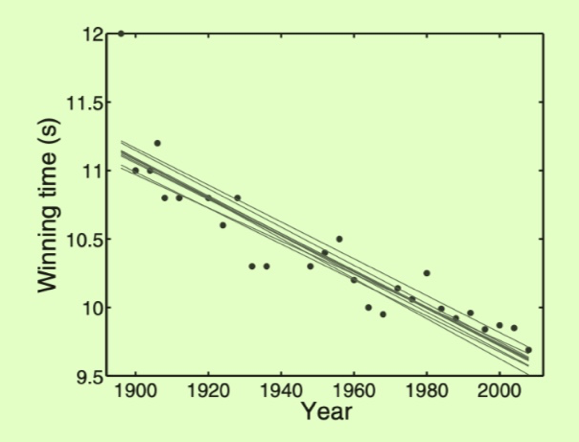Diagonal Elements
• off-diagonal elements: if we were to slightly increase either $w _{0}$ or $w _{1}$, we would have to slightly decrease the other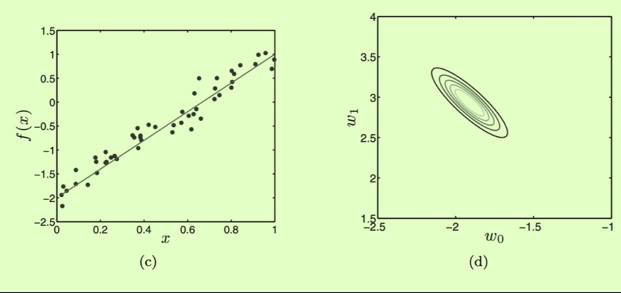Off Diagonal Elements

### Vairability in predictions

If we are quite certain about our prediction, this range might be small; if we are less certain, it might be large. $t _ { \mathrm { new } } = \widehat { \mathbf { w } } ^ { \mathrm { T } } \mathbf { x } _ { \mathrm { new } }$ \begin{aligned} \mathbf { E } _ { p ( \mathbf { t } | \mathbf { X } , \mathbf { w } , \sigma ^ { 2 } ) } \left\{ t _ { \mathrm { new } } \right\} & = \mathbf { E } _ { p ( \mathbf { t } | \mathbf { X } , \mathbf { w } , \sigma ^ { 2 } ) } \{ \widehat { \mathbf { w } } \} ^ { \top } \mathbf { x } _ { \mathrm { new } } \\ & = \mathbf { w } ^ { \top } \mathbf { x } _ { \mathrm { new } } \end{aligned}

$\sigma _ { \mathrm { new } } ^ { 2 } = \operatorname { var } \left\{ t _ { \mathrm { new } } \right\} = \mathbf { E } _ { p ( \mathbf { t } | \mathbf { X } , \mathbf { w } , \sigma ^ { 2 } ) } \left\{ t _ { \mathrm { new } } ^ { 2 } \right\} - \left( \mathbf { E } _ { p ( \mathbf { t } | \mathbf { X } , \mathbf { w } , \sigma ^ { 2 } ) } \left\{ t _ { \mathrm { new } } \right\} \right) ^ { 2 }$

\begin{aligned} \operatorname { var } \left\{ t _ { \text { new } } \right\} & = \mathbf { E } _ { p ( \mathbf { t } | \mathbf { X } , \mathbf { w } , \sigma ^ { 2 } ) } \left\{ \left( \widehat { \mathbf { w } } ^ { \top } \mathbf { x } _ { \text { new } } \right) ^ { 2 } \right\} - \left( \mathbf { w } ^ { \top } \mathbf { x } _ { \text { new } } \right) ^ { 2 } \\ & = \mathbf { E } _ { p ( \mathbf { t } | \mathbf { X } , \mathbf { w } , \sigma ^ { 2 } ) } \left\{ \mathbf { x } _ { \text { new } } ^ { \top } \widehat { \mathbf { w } } \widehat { \mathbf { w } } ^ { \top } \mathbf { x } _ { \text { new } } \right\} - \mathbf { x } _ { \text { new } } ^ { \top } \mathbf { w } \mathbf { w } ^ { \top } \mathbf { x } _ { \text { new. } } \end{aligned}

$\operatorname { var } \left\{ t _ { \mathrm { new } } \right\} = \mathbf { x } _ { \mathrm { new } } ^ { \top } \left( \mathbf { X } ^ { \top } \mathbf { X } \right) ^ { - 1 } \mathbf { X } ^ { \top } \mathbf { E } _ { p ( t | \mathbf { X } , \mathbf { w } , \sigma ^ { 2 } ) } \left\{ \mathbf { t } \mathbf { t } ^ { \top } \right\} \mathbf { X } \left( \mathbf { X } ^ { \top } \mathbf { X } \right) ^ { - 1 } \mathbf { x } _ { \mathrm { new } } - \mathbf { x } _ { \mathrm { new } } ^ { \top } \mathbf { w } \mathbf { w } ^ { \top } \mathbf { x } _ { \mathrm { new } }$

$\operatorname { var } \left\{ t _ { \mathrm { new } } \right\} = \mathbf { x } _ { \mathrm { new } } ^ { \top } \left( \mathbf { X } ^ { \top } \mathbf { X } \right) ^ { - 1 } \mathbf { X } ^ { \top } \left( \sigma ^ { 2 } \mathbf { I } + \mathbf { X } \mathbf { w } \mathbf { w } ^ { \top } \mathbf { X } ^ { \top } \right) \mathbf { X } \left( \mathbf { X } ^ { \top } \mathbf { X } \right) ^ { - 1 } \mathbf { x } _ { \mathrm { new } } - \mathbf { x } _ { \mathrm { new } } ^ { \top } \mathbf { w } \mathbf { w } ^ { \top } \mathbf { x } _ { \mathrm { new } }$

$= \sigma ^ { 2 } \mathbf { x } _ { new } ^ { \top } \left( \mathbf { X } ^ { \top } \mathbf { X } \right) ^ { - 1 } \mathbf { x } _ { new } + \mathbf { x } _ { new } ^ { \top } \mathbf { w } \mathbf { w } ^ { \top } \mathbf { x } _ { new } - \mathbf { x } _ { new } ^ { \top } \mathbf { w } \mathbf { w } ^ { \top } \mathbf { x } _ { new }$ $= \sigma ^ { 2 } \mathbf { x } _ { new } ^ { \top } \left( \mathbf { X } ^ { \top } \mathbf { X } \right) ^ { - 1 } \mathbf { x } _ { new }$ $\Rightarrow$ $\sigma _ { \mathrm { new } } ^ { 2 } = \mathbf { x } _ { \mathrm { new } } ^ { \mathrm { T } } \operatorname { cov } \{ \widehat { \mathbf { w } } \} \mathbf { x } _ { \mathrm { new } }$ $t _ { new } = \mathbf { x } _ { new } ^ { \top } \left( \mathbf { X } ^ { \top } \mathbf { X } \right) ^ { - 1 } \mathbf { X } ^ { \top } \mathbf { t } = \mathbf { x } _ { new } ^ { \top } \widehat { \mathbf { w } }$ $\sigma _ { new } ^ { 2 } = \sigma ^ { 2 } \mathbf { x } _ { new } ^ { \top } \left( \mathbf { X } ^ { \top } \mathbf { X } \right) ^ { - 1 } \mathbf { x } _ { new. }$

A example: $f ( x ) = 5 x ^ { 3 } - x ^ { 2 } + x$, data points sampled from this function and corrupted by Gaussian noise with mean zero and variance 1000.test $t _ { \mathrm { new } } \pm \sigma _ { \mathrm { new } } ^ { 2 }$ for linear, cubic and sixthorder model.

• The increased variability in possible functions caused by the increase in parameter uncertainty is clear for the sixth-order model.
• For all models, the predictive variance increases as we move towards the edge of the data. The model is less confident in areas where it has less data.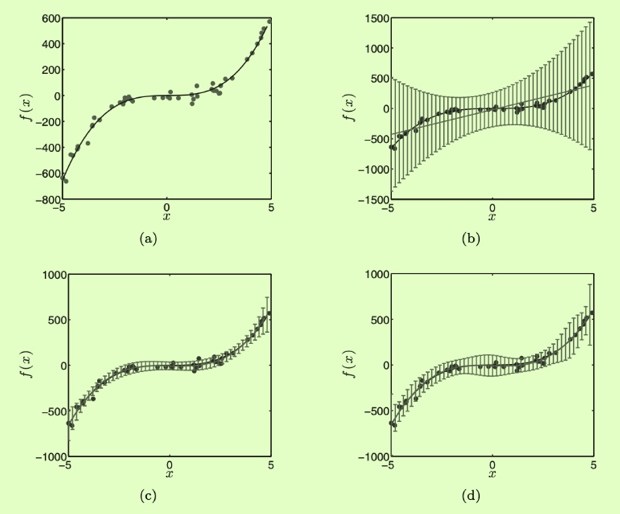All models

code:

import numpy as np
import random
import pylab as plt

def thirdfunc(x):
return 5*x**3 -x**2 + x

if __name__ == '__main__':
x = np.random.uniform(low=-5,high=5,size=(50,))
x.sort()
mu, sigma = 0, 32
s = np.random.normal(mu, sigma, len(x))
y = thirdfunc(x) + s
linex = np.linspace(-5,5,100)
liney = thirdfunc(linex)
plt.subplot(4,2,1)
plt.scatter(x,y,color='blue')
plt.plot(linex,liney, color='red')
X = np.ones_like(x)
inputx = x.reshape(len(x),1)
X = X.reshape(len(X),1)
Y = y.reshape(len(y),1)
for i in range(1,7):
X = np.hstack((X,inputx**i))
w = np.linalg.solve(np.dot(X.T,X) ,np.dot(X.T,Y))
print("estimate sigma'2: ", (Y.T @ Y-Y.T@X@w)/(len(X)))
sigma = (Y.T @ Y-Y.T@X@w)/(len(X))
#plt.figure()
plt.subplot(4, 2, i+1)
#plt.plot(x,X@w)
title = str(i) + " rd"
plt.title(title)
plt.scatter(x,y,color='blue')
plt.plot(linex,liney, color='red')
xnew = np.linspace(-5,5,100)
xnew = xnew.reshape(len(xnew),1)
xerror = np.ones_like(xnew)
for m in range(1,i+1):
xerror = np.hstack((xerror,xnew**m))
inverse = np.linalg.inv(X.T@X)
newsigma = sigma*xerror@inverse@xerror.T
newsigma = np.diag(newsigma)
plt.errorbar(xnew,xerror@w,yerr=newsigma)
#w = np.linalg.solve(A,B)
plt.show()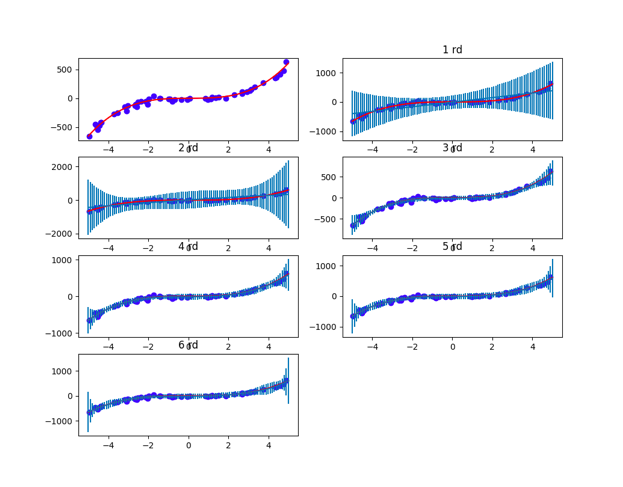Simulation results

Welcome to share or comment on this post: